Envelope
by
Frances LeVangia

Paper size:
This envelope can be folded from a square or a rectangle.

Using the folowing formula, you can calculate what size paper
you need to start with in order to fold an envelope of a certain size.

a = hight of finished envelope
b = width of finished envelope
A = hight of paper
B = width of paper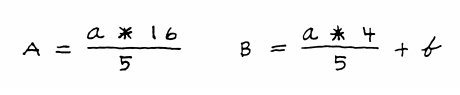For example:
If you want the finished envelope to be 3 inches by 5 inches (a = 3  and b = 5),
then

A = (a x 16) / 5 = (3 x 16) / 5 = 9.6 inches

B = ((a x 4) / 5 ) + b = ((3 x 4) / 5 ) + 5 = 7.4 inches

So, the starting sheet of paper should be 9.6 inches high and 7.4 inches wide.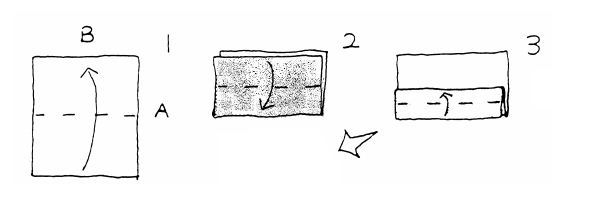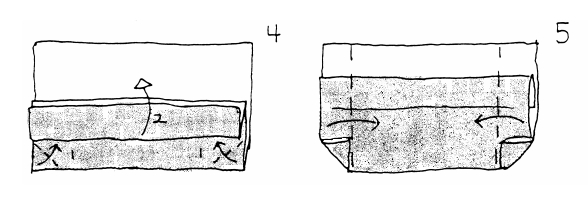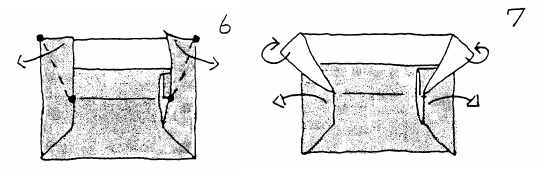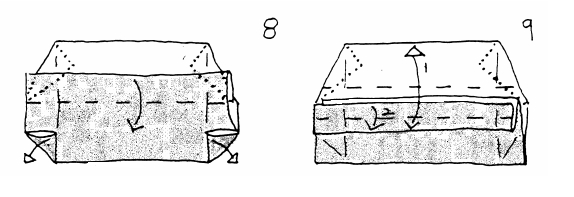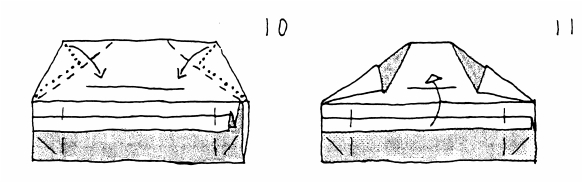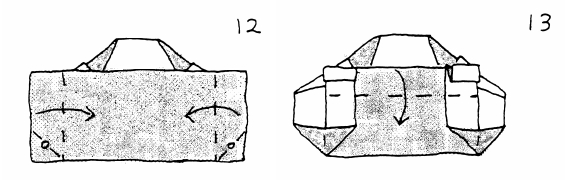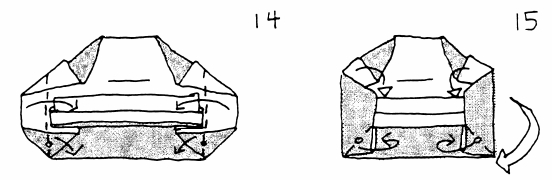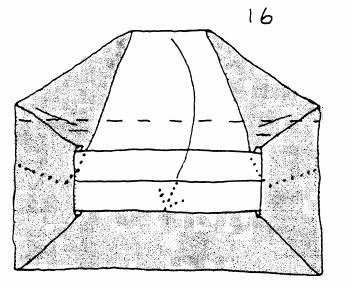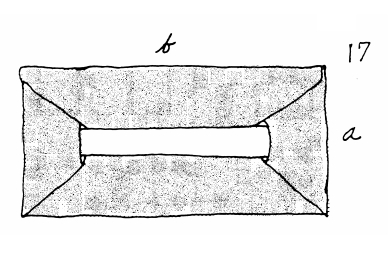( Feel free to teach this model and hand out the diagrams! )

Back to Diagrams
Mette Units Home

All graphics are © Mette Pederson, and may not be used without her permission.